Disclosure: This post may contain affiliate links, meaning when you click the links and make a purchase, we receive a commission.

# How to Find Correlation between Two Variables in Excel

Correlation is a statistical measure that indicates, whether there is a relationship between two variables. Correlation measures the strength of the relationship between two variables, as well as whether or not there is a positive or negative relationship between the two variables.

The discussion of correlation is prevalent in many financial sectors. Financial papers and analysts often evaluate the correlation between the price of gold and let’s say a certain stock.

Positive correlation basically means that as one variables increases, so does the other, whereas a negative correlation refers to a situation where as one variable increases, the other decreases.

One can use either the CORREL Function or the Analysis ToolPak to deliver the correlation coefficient between two variables.

The values for the correlation coefficient r fall in the range of +1.0 to -1.0, depending on the strength of the relationship between the two variables. An r of 0 indicates that there is no relationship between the two variables. An r of +1.0 describes a perfect correlation between two variables whereas an r of -1.0 describes a perfect negative correlation.

There is one main issue to note with the correlation coefficient and that is that it should not be used to denote a cause and effect relationship. For example, smoking causes an increase in the risk of lung cancer, whereas smoking and alcoholism are correlated (the one does not cause the other in this scenario). It is very important to note that there may be another variable affecting the relationship between two variables and therefore not use correlation as a causation indicator.

What do I mean by all of this? , well, let’s get started with a simple example.

## Introduction

A store manager working at a hypothetical department store is looking at the relationship between the monthly number of free complimentary makeovers given by the beauty department and the monthly sales of makeup sets in that specific department. The source data is shown below.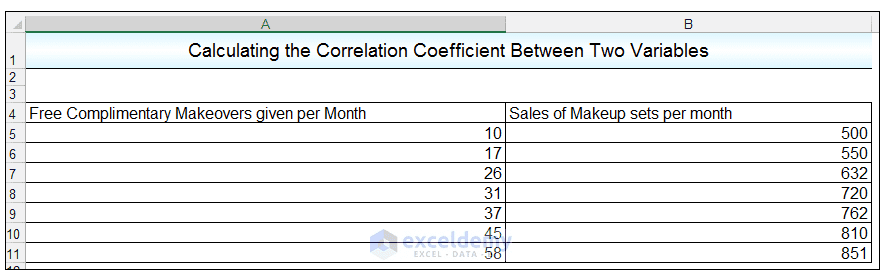## We are going to use the CORREL function first in order to calculate the correlation coefficient

1) In a cell in the worksheet, we enter the formula

=CORREL (A5: A11, B5: B11)

Where A5: A11 is the first data array, namely the free complimentary makeovers given per month, array two refers to the range B5: r valueB11 which is the number of makeup sets sold per month over the given period.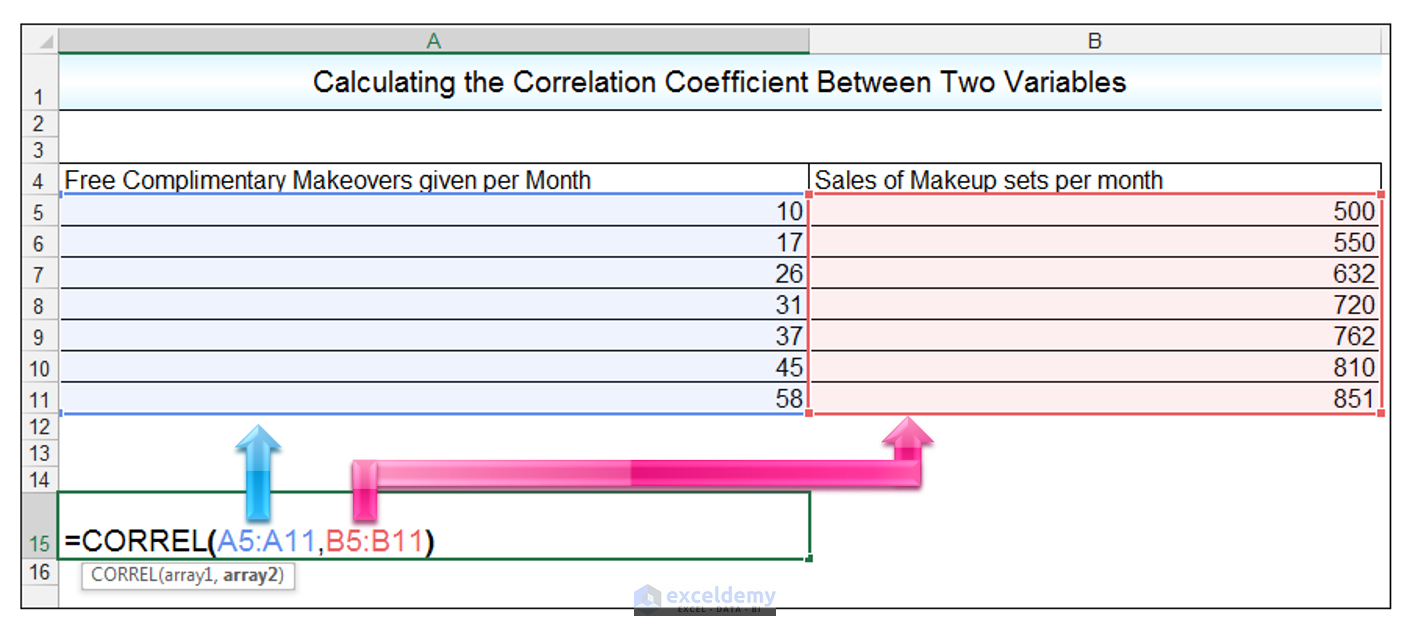2) Pressing CTRL-ENTER results in a correlation coefficient or r-value of 0.973 being returned.

## Using the Correlation option in the Analysis Tool Pak Add-in

We are going to use the Analysis ToolPak Add-in to calculate the correlation coefficient

1) Go to Data -> Analysis -> Data Analysis.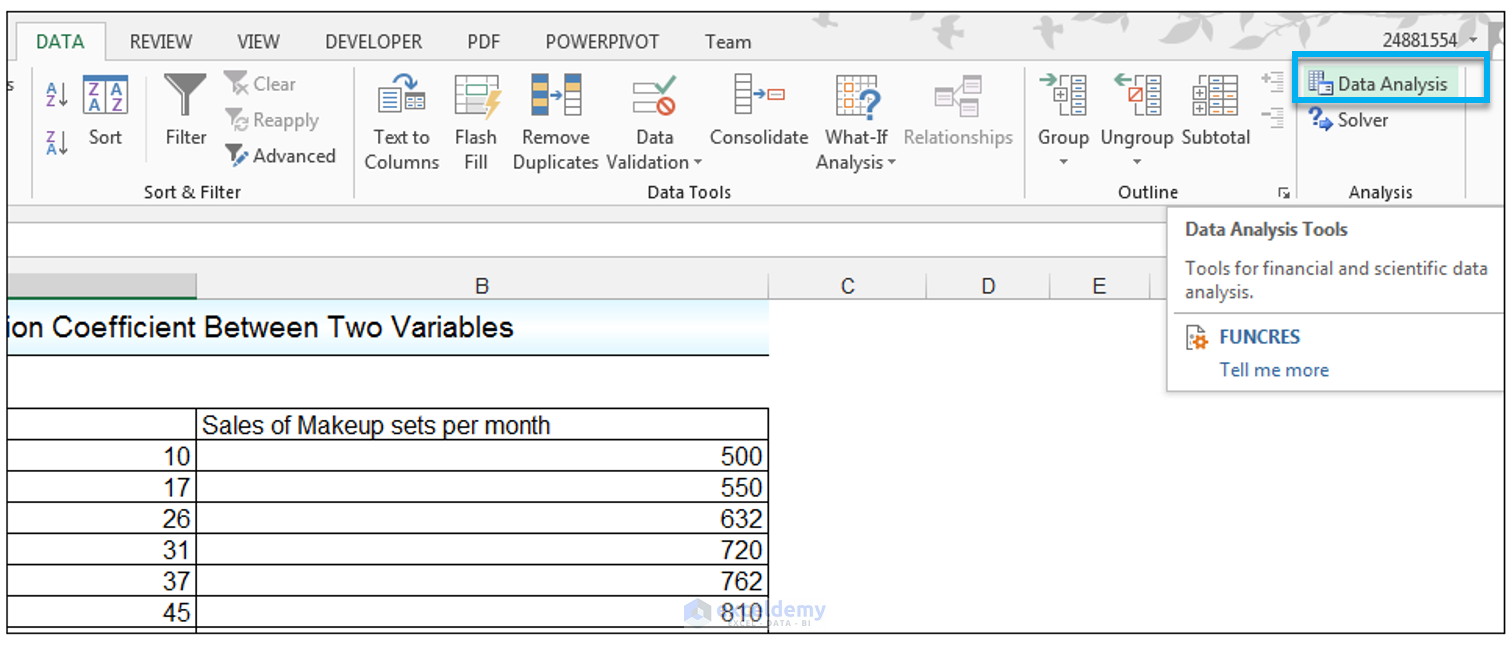2) Select Correlation and then click Ok as shown.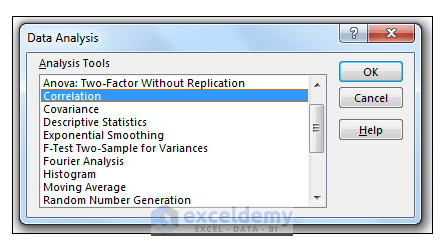3) Select range \$A\$4: \$B\$11 containing the data, as the input range. Make sure Labels in the first row is checked. For the output, range choose cell \$A\$16 as shown below.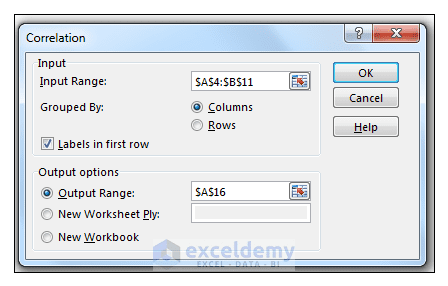4) Click Ok.

5) We get the same result as when using the CORREL function.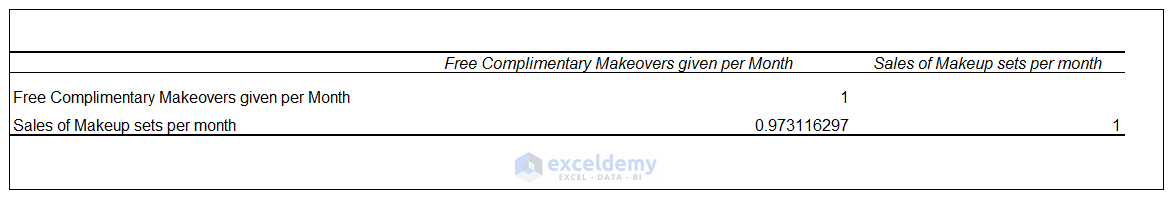Taking our results in context, we can see that there is a strong positive correlation between the free complimentary makeovers given per month and the sales of makeup sets per month as indicated by the correlation coefficient r-value of 0.973, which is very close to 1.

However, we cannot use these results to indicate a cause and effect relationship, since the increase in sales of makeup sets per month may also be influenced by other factors such as an increase in ads in print media advertising the makeup sets for example.

## Conclusion

Correlation and the correlation coefficient is often discussed in many financial sectors such as the stock market and investment portfolio analysis, therefore it helps to have an understanding of what is being discussed.